stat_coverage {ggbio} R Documentation

## Calculate coverage

### Description

Calculate coverage.

### Usage

```# for GRanges
## S4 method for signature 'GRanges'
stat_coverage(data, ..., xlim, xlab, ylab, main,
facets = NULL, geom = NULL)
# for GRangesList
## S4 method for signature 'GRangesList'
stat_coverage(data, ..., xlim, xlab, ylab, main,
facets = NULL, geom = NULL)

# for Bamfile
## S4 method for signature 'BamFile'
stat_coverage(data, ..., maxBinSize = 2^14,
xlim, which, xlab, ylab,
main, facets = NULL, geom = NULL,
method = c("estimate", "raw"),
space.skip = 0.1, coord = c("linear", "genome"))
```

### Arguments

 `data` A `GRanges` or `data.frame` object. `...` Extra parameters such as aes() passed to `geom_rect`, `geom_alignment`, or `geom_segment`. `xlim` Limits for x. `xlab` Label for x `ylab` Label for y `main` Title for plot. `facets` Faceting formula to use. `geom` The geometric object to use display the data. `maxBinSize` maxBinSize. `method` 'estimate' for parsing estimated coverage(fast), 'raw' is slow and parse the accurate coverage. `which` `GRanges` which defines region to subset the results. `space.skip` used for coordinate genome, skip between chromosomes. `coord` coordinate system.

A 'Layer'.

Tengfei Yin

### Examples

``````library(ggbio)
## ======================================================================
##  simmulated GRanges
## ======================================================================
set.seed(1)
N <- 1000
library(GenomicRanges)
gr <- GRanges(seqnames =
sample(c("chr1", "chr2", "chr3"),
size = N, replace = TRUE),
IRanges(
start = sample(1:300, size = N, replace = TRUE),
width = sample(70:75, size = N,replace = TRUE)),
strand = sample(c("+", "-", "*"), size = N,
replace = TRUE),
value = rnorm(N, 10, 3), score = rnorm(N, 100, 30),
sample = sample(c("Normal", "Tumor"),
size = N, replace = TRUE),
pair = sample(letters, size = N,
replace = TRUE))
ggplot(gr) + stat_coverage()
``````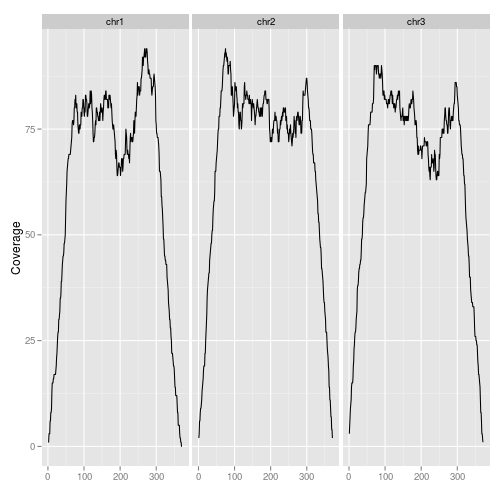``````ggplot() + stat_coverage(gr)
````````````ggplot(gr) + stat_coverage(geom = "point")
``````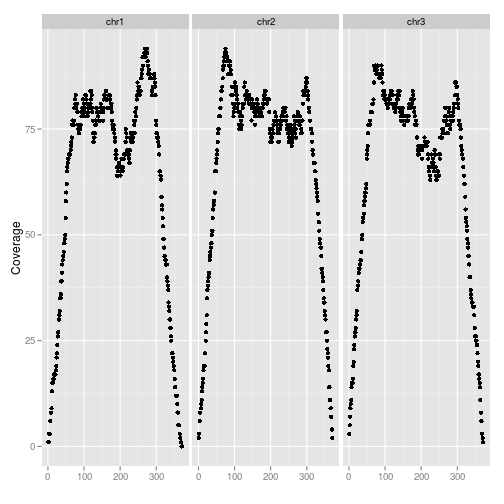``````ggplot(gr) + stat_coverage(geom = "area")
``````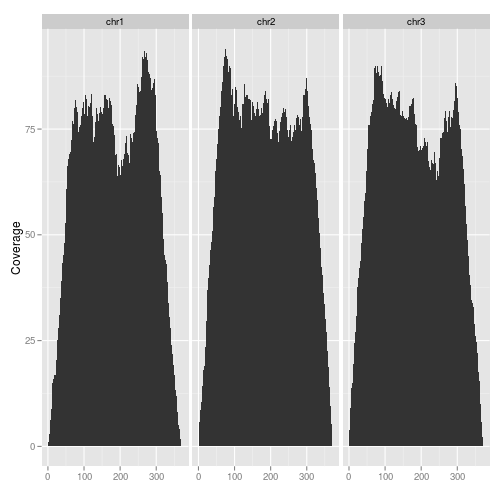``````ggplot(gr) + stat_coverage(aes(y = ..coverage..), geom = "histogram")
``````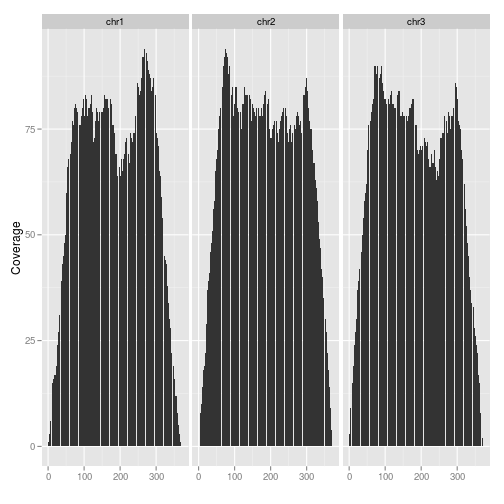``````ggplot(gr) + stat_coverage(aes(y = ..coverage..)) + geom_point()
``````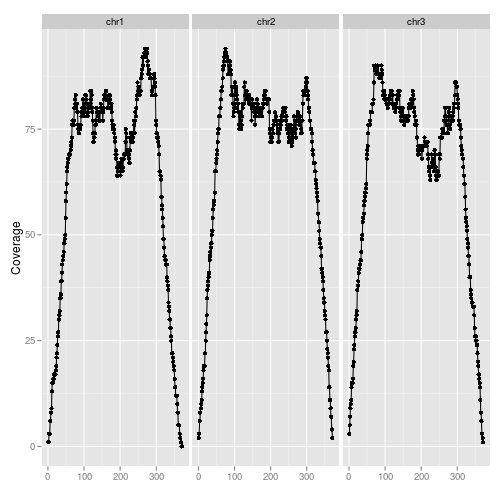``````## for bam file
## TBD
``````

[Package ggbio version 1.5.20 ]